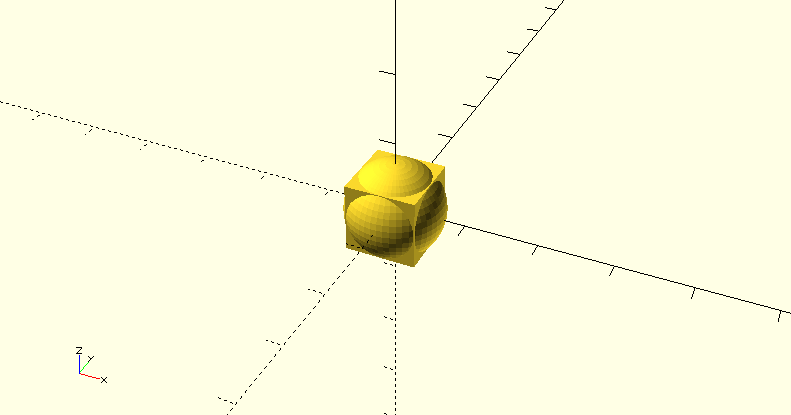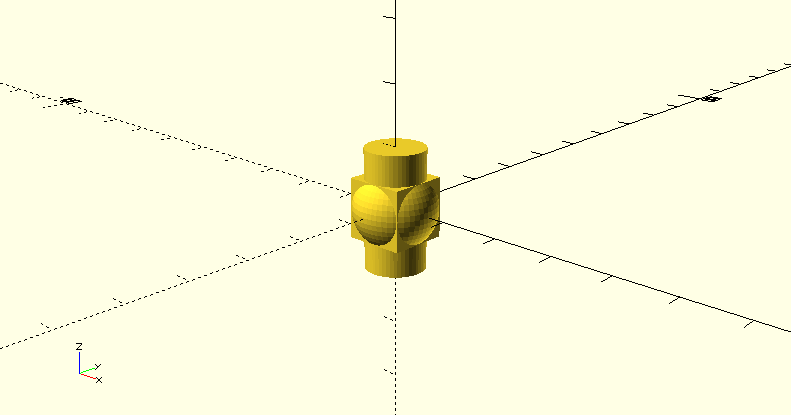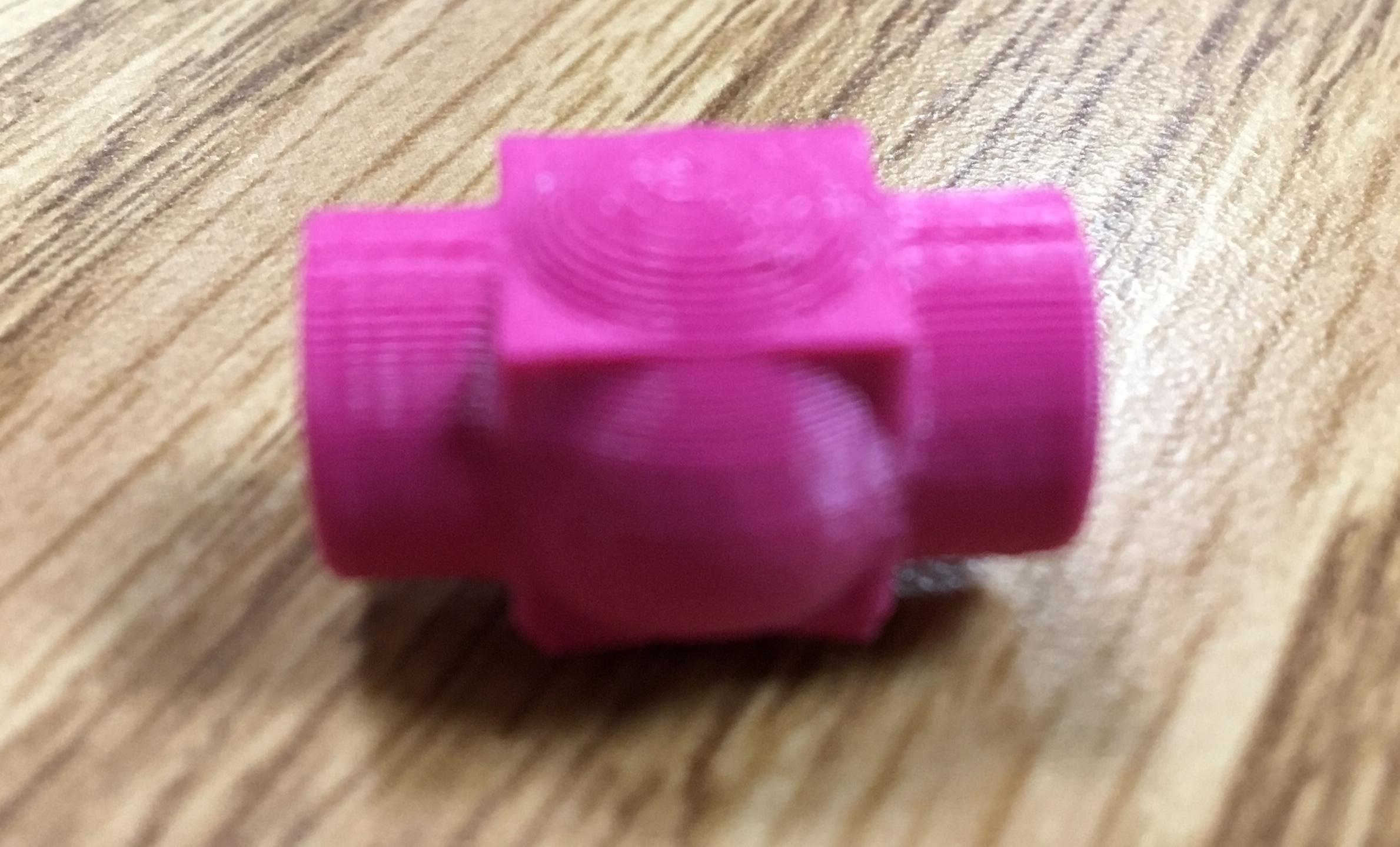When working on this project, I quickly realized that I was not going to be successful at coding. The initial design that I made with code was simply a sphere inside of a cube. I wanted to make it more complicated, but could not figure out how.### Leveled-up Design

Our next requirement for this project was to make the code more complicated. As previously stated, I was not able to figure out how to successfully code. Therefore, when attempting to make the code more complicated I figured out how to insert a cylinder into my design. The next code I wanted to insert was a second cylinder to make a hole in the first cylinder. Unfortunately, I was not able to figure this out even though I looked up some code that might have helped me.First Design Code:

```\$fn=50;

cube(10, center = true);
sphere(7, center = 1);```

Second Design Code:

```\$fn=50;

cube(10, center = true);
sphere(7, center = 1);

//cylinder with cylindrical hole through it
difference(){cylinder(d=10, h=20, center=true); //outside
rotate([90,0,0]) cylinder(d=8, h=20, center=true); //hole }```

David code